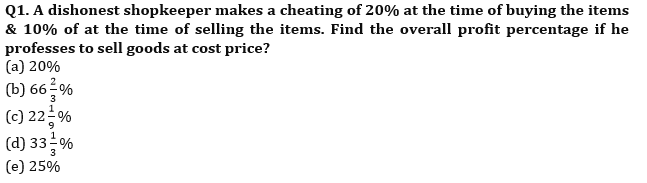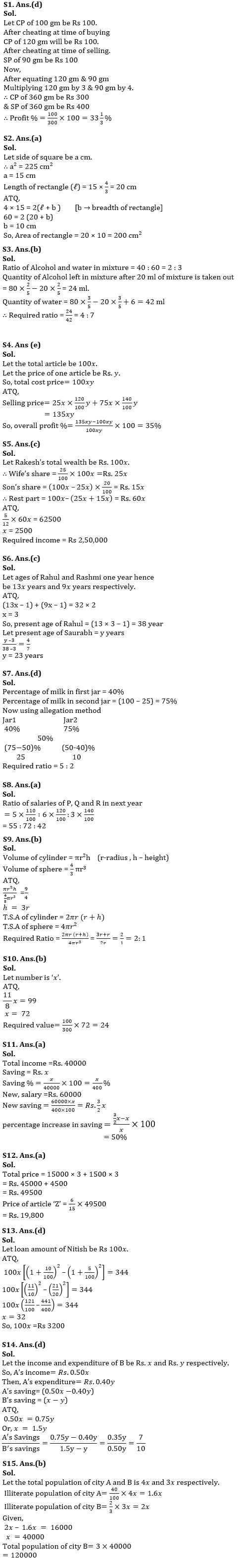Latest Banking jobs   »

# Quantitative Aptitude Quiz For IBPS RRB PO/Clerk Prelims 2022- 11th July

.                                                                            .Q2. Perimeter of rectangle is equal to the perimeter of square which area is 225 cm² and length of rectangle is 33 ⅓% more than the side of a square then find the area of rectangle?
(a) 200 cm²
(b) 140 cm²
(c) 168 cm²
(d) 248 cm²
(e) 348 cm²

Q3. In a mixture of alcohol and water, the proportion of alcohol is 40%. If from the 80 ml mixture, 20 ml of mixture is taken out and 6 ml of pure water is added to the mixture then find the respective ratio of alcohol and water in the new mixture?
(a) 7 : 4
(b) 4 : 7
(c) 4 : 3
(d) 3 : 4
(e) 7 : 3

Q4. A person buys some articles. He sold 25% of articles at 20% profit and remaining at 40% profit. Find the overall profit/loss percentage?
(a) 20%
(b) 50%
(c) 25%
(d) 40%
(e) 35%

Q5. Rakesh distributed 25% of his monthly income to his wife, 20% of remaining to his son and rest to his daughter and a charitable trust in the ratio of 7 : 5. If amount given to charitable trust was Rs.62500 then find Rakesh’s monthly income (in Rs.)?
(a) 5,40,000
(b) 3,40,000
(c) 2,50,000
(d) 3,50,000
(e) 4,50,000

Q6. The ratio of ages of Rahul to Saurabh 3 year ago was 7: 4 and ratio of ages of Rahul and Rashmi one year hence will be 13: 9. If average of present age of Rahul and Rashmi is 32 years, then find the present age of Saurabh?
(a) 25 years
(b) 20 years
(c) 23 years
(d) 18 years
(e) 33 years

Q7. Two Jar contains mixture of milk and water. First jar contains 40% milk and second jar contains 25% water. In what respective ratio these two mixtures are mixed so that new mixture contains 50% milk?
(a) 2 : 7
(b) 7 : 5
(c) 5 : 7
(d) 5 : 2
(e) 2 : 5

Q8. Present salaries of P, Q and R are in the ratio of 5 : 6 : 3. In the next year, their salaries increases by 10%, 20% and 40% respectively, find the ratio of their salaries in the next year?
(a) 55: 72: 42
(b) 25: 71: 32
(c) 82: 15: 42
(d) 21: 53: 123
(e) 3: 8:11

Q9. If ratio between volume of a cylinder and volume of sphere is 9: 4, then find the respective ratio between total surface area of cylinder to total surface area of sphere? [Radius of sphere = Radius of cylinder]
(a) 1: 2
(b) 2: 1
(c) 4 : 1
(d) 3 : 2
(e) 7 : 2

Q10. What is the 33 ⅓% of a number, whose 11/8 th is 99?
(a) 36
(b) 24
(c) 48
(d) 12
(e) 60

Q11. If Anil’s income is Rs 40,000, then he saves Rs. x . If his salary is Rs 60,000 then at what percent his saving increases, such that saving percent of income remains same?
(a) 50%
(b) 80%
(c) 40%
(d) 75%
(e) 60%

Q12. The average price of three different articles X, Y and Z is Rs. 15000. If the price of each article is increased by Rs. 1500 then the ratio of these article becomes 4 : 5 : 6 respectively. Find the price of article ‘Z’ after increment in price?
(a) Rs. 19,800
(b) Rs. 18,000
(c) Rs. 23,500
(d) Rs. 20,000
(e) Rs. 16,500

Q13. In new year offer ABC bank reduced interest rate from 10% to 5% C.I. P.A. Due to this offer Nitish has to pay Rs. 344 less interest in two years. Find the loan amount of Nitish?
(a) Rs. 1,800
(b) Rs. 2,400
(c) Rs. 4,400
(d) Rs. 3,200
(e) Rs. 2,000

Q14. A’s income is 50% of B’s income and A’s expenditure is 40% of B’s expenditure. If A’s income is 75% of B’s expenditure, then find the respective ratio of A’s savings to B’s savings?
(a) 1 : 2
(b) 2 : 5
(c) 5 : 2
(d) 7 : 10
(e) 10 : 7

Q15. The population of two cities A and B are in ratio 4: 3. If 60% and 33 ⅓% of population of city A and city B respectively is literate and the difference between the number of illiterate people of the cities is 16000 then find the total population of city B?
(a) 13000
(b) 12000
(c) 15000
(d) 16000
(e) 25000

Solutions#### Congratulations!Incorrect details? Fill the form again here

•Reasoning Ability Quiz For IBPS RRB PO C...
•Quantitative Aptitude Quiz For IBPS RRB ...
•Reasoning Ability Quiz For IBPS RRB PO C...
•Quantitative Aptitude Quiz For IBPS RRB ...
•Reasoning Ability Quiz For IBPS RRB PO C...
•Reasoning Ability Quiz For IBPS RRB PO C...